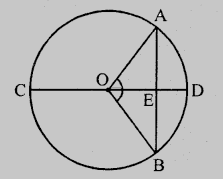# Prove that a diameter of a circle which bisects a chord of the circle also bisects the angle subtended by the chord at the centre of the circle.

To do:

We have to prove that a diameter of a circle which bisects a chord of the circle also bisects the angle subtended by the chord at the centre of the circle.

Solution:

Let in a circle with centre $O, CD$ is the diameter and $AB$ is the chord

which is bisected by diameter at $E$.Join $OA$ and $OB$.

In $\triangle OAE$ and $\triangle OBE$,

$OA = OB$             (Radii of the circle)

$OE = OE$           (Common)

$AE = EB$          (Given)

Therefore, by SSS axiom,

$\triangle OAE \cong \triangle OBE$

This implies,

$\angle AOE = \angle BOE$         (CPCT)

Therefore, the diameter bisects the angle subtended by the chord.

Hence proved.

Updated on: 10-Oct-2022

26 Views